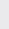Course Content

0/4

0/3

0/1

0/2

0/1

0/4

0/3

0/5

0/3

0/5

0/5

0/1

0/6

0/2

0/4

0/5

12.4 Angle Between Two Lines 两条直线的夹角

(1)
If A(0, 1) and B(4, 3), find the equation of the line passing through B, making 45with AB and cut the y-axis at the negative part.
If this line cut the x-axis at C, find the area ofABC.

y = 3x – 9，5
(2)
Given that the equation of the hypotenuse of an isosceles right angle triangle is 3x – y + 1 = 0, the coordinate of the right angle is (6, 1), find the equations of the other two sides.

y = -2x + 13 , y =x – 2
(3)
In an isoscelesABC, AB = AC also the equation of AB and BC are 2x – y + 2 = 0 and x + y + 1 = 0 respectively. The line AC passes through the point (4, 0), find the equation of AC.

y =x – 2
(4)
In an equilateralABC, A(2, 3) and equation BC is x + y = 0. Find the equations of the other two sides.